## CIE (2012) 2-deg XYZ “physiologically-relevant” colour matching functions

### Data key

Columns

1. Wavelength (nm)
2.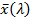3.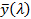4.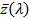Notes

These 2-deg colour matching functions are linear transformations of the 2-deg cone fundamentals of Stockman & Sharpe (2000), ratified by the CIE (2006) as the new physiologically-relevant fundamental CIE CMFs.
The transformation produces CMFs similar to the original 1931,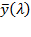and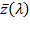CMFs, a colour space still favoured by many engineers and scientists.

The transformation is given by: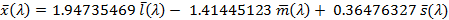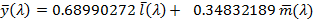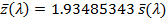where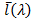,andare the Stockman & Sharpe (2000) or CIE (2006) 2-deg cone fundamentals, which are tabulated here.

The derivation of this transformation is relatively straightforward. TheCMF is the luminous efficiency function originally proposed by Sharpe et al. (2005), but then corrected (Sharpe et al., 2011).  TheCMF is the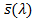cone fundamental originally proposed by Stockman, Sharpe & Fach (1999) scaled to have an equal integral to theCMF for an equal energy white. The definition of theCMF owes much to the work of Jan Henrik Wold for the CIE TC 1-36 committee. Its derivation depends on the following conditions:

1.      Like the other CMFs, the values ofare all positive.

2.      The integral offor an equal energy white is identical to the integrals forand.

3.      The coefficients of the transformation that yieldsare optimized to minimize the Euclidian differences between the resulting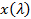,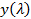and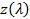chromaticity coordinates and the CIE 1931,andchromaticity coordinates.

Following the usual convention,,andare given in units of energy.

The proposed CIE standard is also concerned about defining the precision of the CMFs. Thus, the coefficients of the transformation are defined to 8 decimal places (as in the equations above), the CMFs themselves are calculated from the cone fundamentals given in linear (energy) units to 9 significant figures (given here), and the results in linear (energy) units are tabulated to 7 significant figures.  In addition, the standard is strictly only for the CMFs tabulated at 1-nm steps given here, since only at this step-size are the integrals of the three CMFs precisely equal.

The functions are provided here at 0.1, 1 and 5 nm steps.

Please note that these are proposals that have yet to be ratified by the full TC 1-36 committee or by the CIE.

References

CIE. (1932). Commission Internationale de l’Éclairage Proceedings, 1931. Cambridge: Cambridge University Press.

Stockman, A., Sharpe, L. T., & Fach, C. C. (1999). The spectral sensitivity of the human short-wavelength cones. Vision Research, 39, 2901-2927.

Stockman, A., & Sharpe, L. T. (2000). Spectral sensitivities of the middle- and long-wavelength sensitive cones derived from measurements in observers of known genotype. Vision Research, 40, 1711-1737.

Sharpe, L. T., Stockman, A., Jagla, W. & Jägle, H.(2005). A luminous efficiency function, V*(λ), for daylight adaptation. Journal of Vision, 5, 948-968.

CIE (2006). Fundamental chromaticity diagram with physiological axes.Parts 1 and 2. Technical Report 170-1. Vienna: Central Bureau of the Commission Internationale de l' Éclairage.

Sharpe, L. T., Stockman, A., Jagla, W. & Jägle, H. (2011). "A luminous efficiency function, V*(λ), for daylight adaptation: a correction." Color Research & Application, 36, 42-46.